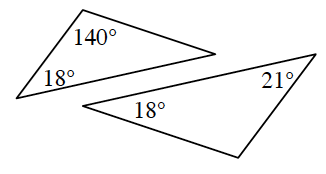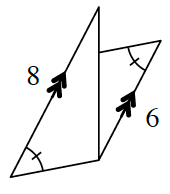### Home > CCG > Chapter 6 > Lesson 6.1.3 > Problem6-23

6-23.

For each pair of triangles below, decide if the pair is similar, congruent or neither. Justify your conclusion with a flowchart or the reasons why the triangles cannot be similar or congruent. Assume that the diagrams are not drawn to scale. Redraw and label the diagrams as needed.

1.Each triangle has a missing angle.
Use the Triangle Angle Sum Theorem to find the measure of the angles.

Not similar.
There are not three pairs of corresponding angles that are congruent.

1.What kind of angles do parallel lines create?
Does this make the triangles similar?